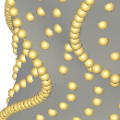CGAL 4.7 - Scale-Space Surface Reconstruction
Scale-Space Surface Reconstruction ReferenceThijs van Lankveld
This method allows to reconstruct a surface that interpolates a set of 3D points. This method provides an efficient alternative to the Poisson surface reconstruction method. The main difference in output is that this method reconstructs a surface that interpolates the point set (as opposed to approximating the point set). How the surface connects the points depends on a scale variable, which can be estimated semi-automatically.

Introduced in: CGAL 4.6
BibTeX: cgal:ssr3-15b
License: GPL
Depends on: 3D Alpha Shapes, dD Spatial Searching, eigenvector solver such as Eigen 3.1.2 (or greater)

## Concepts

• WeightedApproximation_3

## Classes

• CGAL::Scale_space_surface_reconstruction_3<Gt,FS,Sh,wA,Ct>
• CGAL::Weighted_PCA_approximation_3<Kernel>

Concepts

Classes